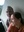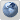Coffeehouse for desis
Would you like to react to this message? Create an account in a few clicks or log in to continue.

# On the importance of zero (0) and decimal system (using 9 + 1 = 10) to mathematics, science and business##On the importance of zero (0) and decimal system (using 9 + 1 = 10) to mathematics, science and business

The Map on Mathematics (Ref. 1) unfortunately does not mention the greatest discovery / invention in mathematics (0 or zero / shunya) and its incorporation (as relation 9 + 1 = 10) to create decimal system which had led mankind on the path to progress in mathematics (including number theory), science and business due to the ability of decimal system to define numbers elegantly, uniquely and irrespective of size in terms of only 10 digits (0-9), thus making it also possible and easy to perform mathematical operations (including additions, subtractions, multiplications and divisions) using numbers.

Furthermore, binary system (using 0, 1, and based on 1 + 1 = 10) and hexadecimal system (using 0, 1, 2....,9, A, B, C, D, E, F, and based on F + 1 = 10), at the heart of computers recently, have their roots (similarities etc.) in the ancient decimal system (using 0, 1, ..., 9, and based on 9 +1 = 10).

Let's express as example in the following, in different numbering systems, Ramanujan number 1729 (the sum of 9-cubed and 10-cubed, i.e. 1729 = 9^3 + 10^3, in decimal system):

(a) Hexadecimal system (using 0, 1,..., 9, A for 10, B for 11, C for 12, D for 13, E for 14, F for 15, and the relation F + 1 =10) transforms 1729 (decimal) as 6C1 (hexadecimal);

(b) Binary system (using 0, 1, and the relation 1 + 1 = 10) transforms 1729 (decimal) as 110011000001 (binary); and

(c) Roman numerals system (lacking 0, and using I for 1, V for 5, X for 10, L for 50, C for 100, D for 500, M for 1000) transforms 1729 (decimal) as MDCCXXIX (Roman numerals).

As can be seen above, there is no way for efficient expres​sion(writing) and operation (addition etc.) of numbers in the zero-less numbering systems (e.g. Roman numerals, lacking 0 explicitly and not using 10 implicitly). Furthermore, the idea and identification of prime numbers (in number theory etc.) would not occur and advance if zero (0) had not been invented and incorporated (as 10) giving rise to decimal system initially which, unlike zero-less systems (Roman numerals etc.), made it possible and easy to multiply and divide numbers leading to the discovery of primes.

References

(1) Kevin Harnett / Quanta Magazine, "The Map of Mathematics," Feb. 13, 2020, https://www.quantamagazine.org/the-map-of-mathematics-20200213/Seva Lamberdar

Posts : 6555
Join date : 2012-11-29##Re: On the importance of zero (0) and decimal system (using 9 + 1 = 10) to mathematics, science and business

There is no question about the extraordinary importance and enormity of zero (0) and the decimal system (using 0 explicitly and implicitly as 10) in everything (especially mathematics, science and business) that requires counting, measuring and calculating using numbers while carrying out mathematical operations (addition, subtraction, multiplication and division).

Zero (0) also had led to the concept of negative numbers (less than 0), in addition to the positive numbers (greater than 0) previously.

Similarly, the efficient use of numbers less than 1 (including fractions) was the result of decimal system (using 0 explicitly and implicitly as 10), due mainly to its ability and efficacy in multiplying and dividing numbers easily, quickly and accurately.Seva Lamberdar

Posts : 6555
Join date : 2012-11-29Permissions in this forum:
You cannot reply to topics in this forum